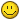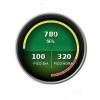## Recommended Posts

Hi!

I use the Angular Gauge to show some values in pattern of hours and send this values for Fusico Chart in milliseconds.

I try to apply a number format to show my value in this pattern 05:04 (mm:ss), but i don't have success.

My chart is configure on this form:

<... numberScaleValue='60,60' numberScaleUnit=' , ' formatNumberScale='1' scaleRecursively='1' scaleSeparator=':' decimalPrecision='0'

But my hour is show in this pattern 5:4 and i need to show 05:04 (a zero reference).

How i can make this?

Thanks

##### Share on other sitesHi,

Welcome to FusionCharts Forum!You would need to pass the value as a string using <displayValue> attribute .

Hope this helps.##### Share on other sites

Hi Angie, thank you!

In Attach the style of my Gauge.

The red pointer is working as velocimeter respecting thresholds.

But the numbers i can't show converted in hours format, he's lost de zero values.

The propertie displayValue respect the thresholds and make the pointer or is only for visualization?

Thanks!

Hi,

Welcome to FusionCharts Forum!You would need to pass the value as a string using <displayValue> attribute .

Hope this helps.##### Share on other sites

Please Angel, you can help me?

I want to change this data and not have success.

Thanks

Hi Angie, thank you!

In Attach the style of my Gauge.

The red pointer is working as velocimeter respecting thresholds.

But the numbers i can't show converted in hours format, he's lost de zero values.

The propertie displayValue respect the thresholds and make the pointer or is only for visualization?

Thanks!

##### Share on other sitesHi,

Could you please send us the XML code to test it from our end?

##### Share on other sites

Hi,

Could you please send us the XML code to test it from our end?

Hi Angshu! Thank for your help.

Attached is XML:

```<Chart bgColor='000000' bgAlpha='0' upperLimit='".\$thresholds["limite"]."' lowerLimit='0' baseFontColor='FFFFFF' toolTipBorderColor='333333' toolTipBgColor='ffffff' gaugeOuterRadius='100' gaugeOriginX='150' gaugeOriginY='150' gaugeStartAngle='180' gaugeEndAngle='-90' placeValuesInside='0' gaugeInnerRadius='94%25' annRenderDelay='0' gaugeFillMix='' pivotRadius='0' showShadow='0' showBorder='0' autoScale='1' origW='300' origH='300' baseFontSize='20' showToolTip='0' majorTMColor='ffffff' majorTMAlpha='100' majorTMHeight='6' majorTMThickness='1' minorTMColor='ffffff' minorTMAlpha='100' minorTMHeight='6' minorTMThickness='1' showTickValues='0' showGaugeBorder='1' gaugeBorderColor='ffffff' gaugeBorderThickness='1' gaugeBorderAlpha='100' tickValueDistance='5' decimalPrecision='0'>

<dials>
<dial id='valorFinal' value=\"1185000\" borderAlpha=\"0\" bgColor=\"990000\" baseWidth=\"1\" topWidth=\"1\" radius=\"100\" showValue='1' valueY='100'/>
<dial id='picoDia' value=\"778000\" borderAlpha=\"0\" bgColor=\"990000\" baseWidth=\"0\" topWidth=\"0\" radius=\"100\" showValue='1' valueX='105' valueY='180'/>
<dial id='picoHora' value=\"718544\" borderAlpha=\"0\" bgColor=\"990000\" baseWidth=\"0\" topWidth=\"0\" radius=\"100\" showValue='1' valueX='198' valueY='180'/>
</dials>
<colorRange>
<color minValue='0' maxValue='".\$thresholds["minimo"]."' code='009244' alpha='100'/>
<color minValue='".\$thresholds["minimo"]."'  maxValue='".\$thresholds["maximo"]."' code='ffcb06' alpha='100'/>
<color minValue='".\$thresholds["maximo"]."'  maxValue='".\$thresholds["limite"]."' code='d62327' alpha='100'/>
</colorRange>
<styles>
<definition>
<style name='MyFirstFontStyle' type='font' face='Arial' color='FFFFFF' bold='1' />
<style name='TipStyle' type='font' font='Arial' size='14' color='333333' />
</definition>
<application>
<apply toObject='tooltip' styles='TipStyle' />
</application>
</styles>
<annotations>
<annotationGroup xPos='150' yPos='150' showBelow='1' origW='300' origH='300' autoScale='1' scaleText='1' visible='1'>
<annotation type='circle' xPos='0' yPos='0' radius='130' startAngle='0' endAngle='360' fillColor='CCCCCC,555555'  fillPattern='linear' fillAlpha='100,100'  fillRatio='50,50' fillAngle='-45'/>
<annotation type='circle' xPos='0' yPos='0' radius='125' startAngle='0' endAngle='360' fillColor='555555,cccccc'  fillPattern='linear' fillAlpha='100,100'  fillRatio='50,50' fillAngle='-45'/>
<annotation type='circle' xPos='0' yPos='0' radius='120' startAngle='0' endAngle='360' fillColor='222222,000000'  fillPattern='linear' fillAlpha='100,100'  fillRatio='30,70' fillAngle='-90'/>
<annotation type='circle' xPos='0' yPos='0' radius='110' startAngle='0' endAngle='360' fillColor='0d8b44,98c93c'  fillPattern='linear' fillAngle='270' fillRatio='50,50'/>
<annotation type=\"circle\" xPos=\"0\" yPos=\"-16\" radius=\"90\" fillPattern=\"linear\" fillColor=\"000000,ffffff\" fillAlpha=\"15,0\" fillRatio=\"50,50\" fillAngle=\"270\"/>
<annotation type='circle' xPos='0' yPos='0' radius='110' startAngle='355' endAngle='185' fillColor='000000' fillAngle='270' fillAlpha='40' fillRatio='50'/>
<annotation type='line' xPos='0' yPos='10' toYPos='70' color='acc396' thickness='1'/>
<annotation type='text' label='".\$dados["label"]."' font='Arial' xPos='0' yPos='-25' align='center' fontcolor='FFFFFF' fontSize='14' isBold='1'/>
<annotation type='text' label='' font='Arial' xPos='-7' yPos='45' align='right' vAlign='left' fontcolor='FFFFFF' fontSize='12' isBold='1'/>
<annotation type='text' label='' font='Arial' xPos='7' yPos='45' align='left' vAlign='left' fontcolor='FFFFFF' fontSize='12' isBold='1'/>
</annotationGroup>
<annotationGroup xPos='150' yPos='150' showBelow='1' origW='300' origH='300' autoScale='1' scaleText='1' id='statusRed' visible='0'>
<annotation type='circle' xPos='0' yPos='0' radius='130' startAngle='0' endAngle='360' fillColor='CCCCCC,555555'  fillPattern='linear' fillAlpha='100,100'  fillRatio='50,50' fillAngle='-45'/>
<annotation type='circle' xPos='0' yPos='0' radius='125' startAngle='0' endAngle='360' fillColor='555555,cccccc'  fillPattern='linear' fillAlpha='100,100'  fillRatio='50,50' fillAngle='-45'/>
<annotation type='circle' xPos='0' yPos='0' radius='120' startAngle='0' endAngle='360' fillColor='222222,000000'  fillPattern='linear' fillAlpha='100,100'  fillRatio='30,70' fillAngle='-90'/>
<annotation type='circle' xPos='0' yPos='0' radius='110' startAngle='0' endAngle='360' fillColor='ef4937,860a0c'  fillPattern='linear' fillAngle='270' fillRatio='50,50'/>
<annotation type=\"circle\" xPos=\"0\" yPos=\"-16\" radius=\"90\" fillPattern=\"linear\" fillColor=\"000000,ffffff\" fillAlpha=\"15,0\" fillRatio=\"50,50\" fillAngle=\"270\"/>
<annotation type='circle' xPos='0' yPos='0' radius='110' startAngle='355' endAngle='185' fillColor='000000' fillAngle='270' fillAlpha='40' fillRatio='50'/>
<annotation type='line' xPos='0' yPos='10' toYPos='70' color='acc396' thickness='1'/>
<annotation type='text' label='".\$dados["label"]."' font='Arial' xPos='0' yPos='-25' align='center' fontcolor='FFFFFF' fontSize='14' isBold='1'/>
<annotation type='text' label='' font='Arial' xPos='-7' yPos='45' align='right' vAlign='left' fontcolor='FFFFFF' fontSize='12' isBold='1'/>
<annotation type='text' label='' font='Arial' xPos='7' yPos='45' align='left' vAlign='left' fontcolor='FFFFFF' fontSize='12' isBold='1'/>
<annotationGroup xPos='150' yPos='150' showBelow='1' origW='300' origH='300' autoScale='1' scaleText='1' id='statusYellow' visible='0'>
<annotation type='circle' xPos='0' yPos='0' radius='130' startAngle='0' endAngle='360' fillColor='CCCCCC,555555'  fillPattern='linear' fillAlpha='100,100'  fillRatio='50,50' fillAngle='-45'/>
<annotation type='circle' xPos='0' yPos='0' radius='125' startAngle='0' endAngle='360' fillColor='555555,cccccc'  fillPattern='linear' fillAlpha='100,100'  fillRatio='50,50' fillAngle='-45'/>
<annotation type='circle' xPos='0' yPos='0' radius='120' startAngle='0' endAngle='360' fillColor='222222,000000'  fillPattern='linear' fillAlpha='100,100'  fillRatio='30,70' fillAngle='-90'/>
<annotation type='circle' xPos='0' yPos='0' radius='110' startAngle='0' endAngle='360' fillColor='feef00,c06816'  fillPattern='linear' fillAngle='270' fillRatio='50,50'/>
<annotation type=\"circle\" xPos=\"0\" yPos=\"-16\" radius=\"90\" fillPattern=\"linear\" fillColor=\"000000,ffffff\" fillAlpha=\"15,0\" fillRatio=\"50,50\" fillAngle=\"270\"/>
<annotation type='circle' xPos='0' yPos='0' radius='110' startAngle='355' endAngle='185' fillColor='000000' fillAngle='270' fillAlpha='40' fillRatio='50'/>
<annotation type='line' xPos='0' yPos='10' toYPos='70' color='acc396' thickness='1'/>
<annotation type='text' label='".\$dados["label"]."' font='Arial' xPos='0' yPos='-25' align='center' fontcolor='FFFFFF' fontSize='14' isBold='1'/>
<annotation type='text' label='' font='Arial' xPos='-7' yPos='45' align='right' vAlign='left' fontcolor='FFFFFF' fontSize='12' isBold='1'/>
<annotation type='text' label='' font='Arial' xPos='7' yPos='45' align='left' vAlign='left' fontcolor='FFFFFF' fontSize='12' isBold='1'/>
</annotationGroup>
<annotationGroup xPos='150' yPos='150' showBelow='1' origW='300' origH='300' autoScale='1' scaleText='1' id='statusGreen' visible='0'>
<annotation type='circle' xPos='0' yPos='0' radius='130' startAngle='0' endAngle='360' fillColor='CCCCCC,555555'  fillPattern='linear' fillAlpha='100,100'  fillRatio='50,50' fillAngle='-45'/>
<annotation type='circle' xPos='0' yPos='0' radius='125' startAngle='0' endAngle='360' fillColor='555555,cccccc'  fillPattern='linear' fillAlpha='100,100'  fillRatio='50,50' fillAngle='-45'/>
<annotation type='circle' xPos='0' yPos='0' radius='120' startAngle='0' endAngle='360' fillColor='222222,000000'  fillPattern='linear' fillAlpha='100,100'  fillRatio='30,70' fillAngle='-90'/>
<annotation type='circle' xPos='0' yPos='0' radius='110' startAngle='0' endAngle='360' fillColor='0d8b44,98c93c'  fillPattern='linear' fillAngle='270' fillRatio='50,50'/>
<annotation type=\"circle\" xPos=\"0\" yPos=\"-16\" radius=\"90\" fillPattern=\"linear\" fillColor=\"000000,ffffff\" fillAlpha=\"15,0\" fillRatio=\"50,50\" fillAngle=\"270\"/>
<annotation type='circle' xPos='0' yPos='0' radius='110' startAngle='355' endAngle='185' fillColor='000000' fillAngle='270' fillAlpha='40' fillRatio='50'/>
<annotation type='line' xPos='0' yPos='10' toYPos='70' color='acc396' thickness='1'/>
<annotation type='text' label='".\$dados["label"]."' font='Arial' xPos='0' yPos='-25' align='center' fontcolor='FFFFFF' fontSize='14' isBold='1'/>
<annotation type='text' label='' font='Arial' xPos='-7' yPos='45' align='right' vAlign='left' fontcolor='FFFFFF' fontSize='12' isBold='1'/>
<annotation type='text' label='' font='Arial' xPos='7' yPos='45' align='left' vAlign='left' fontcolor='FFFFFF' fontSize='12' isBold='1'/>
</annotationGroup>
</annotations>
</Chart>
```